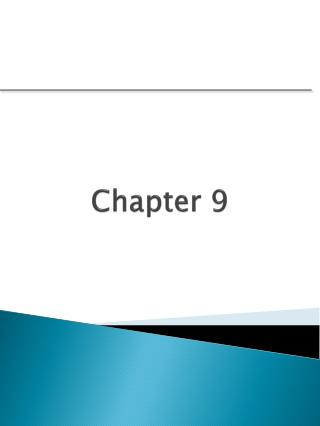# Chapter 9 - PowerPoint PPT PresentationDownload PresentationChapter 9

Chapter 9
Download Presentation## Chapter 9

- - - - - - - - - - - - - - - - - - - - - - - - - - - E N D - - - - - - - - - - - - - - - - - - - - - - - - - - -
##### Presentation Transcript

1. Chapter 9

2. Control Chart Decision Tree Defectives Defects

3. What is a p chart? • An attribute data control chart • Used to chart fraction defective or proportion nonconforming data • The subgroup size can be fixed or it can vary

4. How a p chart is used • Used to establish performance control of any process: • Pass / fail situations • Percent defective per sample • Good for any technical or administrative process

5. 11 step procedure for control charts • Select a process measurement • Stabilize process and decrease obvious variability • Check the gages (10:1, GRR) • Make a sample plan • Setup the charts and process log • Setup the histogram • Take the samples and chart the points • Calculate the control limits and analyze for control • Calculate the capability and analyze for capability • Monitor the process • Continuous Improvement

6. p chart control limits • Chart the points • Sets scales for control chart • Calculate each subgroup’s proportion nonconforming • Plot the proportion nonconforming on the chart • Calculate control limits and analyze for control • Plot your control limits

7. p chart control limits • Calculate the capability and analyze for capability • Capability is based on average defective • Is UCL or LCL within your goal value? • If UCL > USL or LCL < LSL then Cpk<1 • If UCL < USL or LCL > LSL then Cpk>1

8. Advantages of a p chart • Data readily available • Helps prioritize problem areas • Easy to create with fixed and variable subgroup sizes

9. Disadvantages of a p chart • Counts failures - not prevention • Cannot isolate causes of process problems • Variable control limits may be confusing on variable subgroup sizes • Limits hard to construct on variable subgroup sizes

10. p Chart Example This data represents the number of errors found in purchase orders over a 30 week period. 1. Complete a p chart. 2.What can you tell from the data? 3. Complete a np chart. 4.What can you tell from the data? 5. How are these charts different?

11. p chart exercise notes